Open in App
Not now

# Class 10 RD Sharma Solutions – Chapter 15 Areas Related to Circles – Exercise 15.4 | Set 3

• Last Updated : 30 Apr, 2021

### Question 35. In the figure, AB and CD are two diameters of a circle perpendicular to each other and OD is the diameter of the smaller circle. If OA = 7 cm, find the area of the shaded region.

Solution: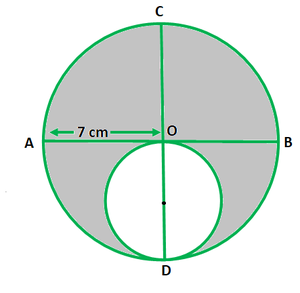Given that,

Radius of larger circle(OA) = 7 cm

Diameter of smaller circle(OD) = 7 cm

So, the radius of smaller circle = 7/2 cm

Now we find the area of the shaded region = Area of large circle – Area of small circle

= π(7)2 – π(7/2)2

= π × 49 – π × (49/4)

=  22/7[49 – 49/4]

= 115.5cm2

Hence, the area of the shaded region 115.5 cm2

### Question 36. In the figure, PSR, RTQ, and PAQ are three semi-circles of diameters 10 cm, 3 cm, and 7 cm respectively. Find the perimeter of the shaded region.

Solution: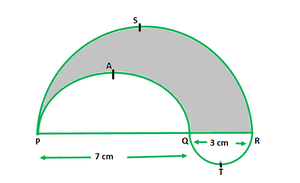Given that,

Diameter of semicircle PSR = 10 cm

So, radius of semicircle PSR, (r1) = 10/2 = 5 cm

Diameter of semicircle RTQ = 3 cm

So, radius of semicircle RTQ, (r2) = 3 cm = 3/2 cm

Diameter of semicircle PAQ = 7 cm

So, radius of semicircle PAQ, (r3) = 7/2 cm

Now we find the perimeter of the shaded region = Length of the arc PAQ +

Length of the arc PSR +

Length of the arc RTQ

= πr1 + πr2 + πr3

= π(r1 + r2 + r3)

= π(5 + 3/2 + 7/2)

= π{(10 + 3 + 7)/2}

= π × 20/2

= 10 π

= 10 × 22/7

= 10 × 3.14

= 31.4 cm

Hence, the perimeter of the shaded region is 31.4 cm

### Question 37. In the figure, two circles with centres A and B touch each other at the point C. If AC = 8 cm and AB = 3 cm, find the area of the shaded region.

Solution: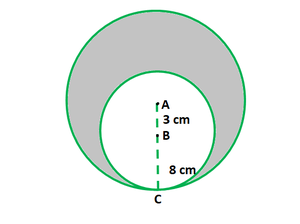Given that,

AC = 8 cm & AB = 3 cm

Here, AC is the radius of the bigger circle and BC is the radius of inner circle.

BC = AC – AB

BC =  8 – 3

BC = 5 cm

Now we find the area of shaded region = Area of bigger circle – Area of inner circle

= πR2 – πr2

= π(R2 – r2)

= 22/7 (82 – 5)

= 22/7 (64 – 25)

= 22/7 × 39

= (22 × 39)/7

= 858/7

= 122.57 cm2

Hence, the area of shaded region is 122.57 cm2

### (ii) the areas of the incircle and the circum- circle of the square.

Solution: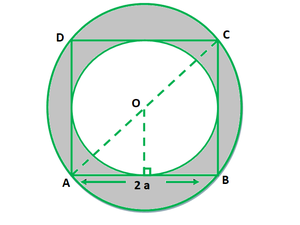Given that,

Side of a square ABCD = 2a

So, the diameter of incircle = side of a square = 2a

Radius of a incircle(r) = Diameter of incircle/2

r = 2a/2

r = a

Also, the diameter of circumcircle = diagonal of a square = √2 side

So, the radius of circumcircle(R) = √2 side/2

R = (√2 × 2a) /2

R = √2a

R = √2a

(i) Ratio of circumferences of inner circle (C1) and circumcircle (C2)

C1 : C2 = 2πr : 2πR

C1 / C2 = 2πr / 2πR

C1 / C2 = r / R

C1 / C2 = a/√2a

C1 / C2 = 1/√2

C1 : C2 = 1 : √2

(ii) Ratio of Areas of inner circle (A1) and circumcircle (A2) :

A1 : A2 = πr2 : πR2

A1 / A2 = πr2 / πR2

A1 / A2 = r2 /R2

A1 / A2 = a2/(√2a)2

A1 / A2 = a2/2a2

A1 / A2 = 1/2

A1 : A2 = 1 : 2

Hence, the ratio of circumferences of inner circle (A1) and circumcircle (A2) is 1 : √2

and Ratio of Areas of inner circle (A1) and circumcircle (A2) is 1 : 2

### (ii) the cost of painting the shaded region at the rate of 25 paise per cm², to the nearest rupee

Solution: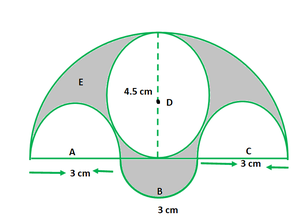Given that,

Three semicircles, A, B and C having diameter 3 cm each, and

another semicircle E having a circle D with diameter 4.5 cm.

(i) Now area of shaded region = Area of the semicircle with diameter 9 cm –

Area of two semicircles with radius 3 cm –

Area of the circle with centre D +

Area of semicircle with radius 3 cm

= 1/2 π(9/2)2 – 2 × 1/2 π(3/2)2 – π(4.5/2)2 + 1/2 π(3/2)2

= 1/2 π(4.5)2 – π(1.5)2 – π(2.25)2 + 1/2 π(1.5)2

= 1/2 π(4.5)2 – π(1.5)2 + 1/2 π(1.5)2 – π(2.25)2

= 1/2 π(4.5)2 –  1/2 π(1.5)2 – π(2.25)2

= 1/2π(4.52 – 1.52) – π 2.252

= 1/2 π (20.25 – 2.25) – π × 5.0625

= 1/2 π(18) – π × 5.0625

= 9π – π 5.0625

= π(9 – 5.0625)

= π × 3.9375

= 22/7 × 3.9375

= 0.5625 × 22

= 12.375 cm2

(ii) Cost of painting 1 cm² Shaded Region = 25 p

Cost of painting 13.275 cm² Shaded Region = 25 p × 13.275

= 309.375 paise

= ₹ 309.375 /100

= ₹ 3 (nearest rupee)

Cost of painting the Shaded Region = ₹ 3.

Hence, the area of the shaded region is12.375 cm2 and the cost of painting the Shaded Region is ₹ 3.

### Question 40. In the figure, ABC is a right-angled triangle, ∠B = 90°, AB = 28 cm and BC = 21 cm. With AC as diameter a semicircle is drawn and with BC as radius, a quarter circle is drawn. Find the area of the shaded region correct to two decimal places.

Solution: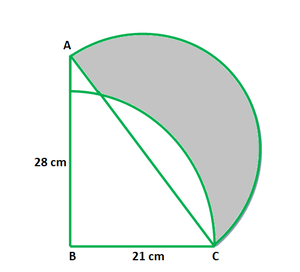Given that,

ABC is a right-angled triangle, ∠B = 90°, AB = 28 cm and BC = 21 cm.

AC as diameter a semicircle is drawn and with BC as radius a quarter circle is drawn

So, the area of triangle = 1/2 x 21 x 28 = 294 cm2

Now in ΔABC,

By using Pythagoras Theorem

AC2 = 282 + 212

AC = √1225

AC = 35

So, the radius of semi-circle = 35/2 = 17.5

Now, the area of semi-circle

= 1/2 x 3.14 x (17.5)2 = 480.8 cm2

Area of quarter circle = 1/4 x 3.14 x 212 = 346.2 cm2

Now we find the area of the shaded region = Area of Semi-circle + Area of triangle ABC –

Area of quarter circle

= 294 + 480.8 – 346.2 = 428.75cm2

Hence, the area of shaded region is 428.75cm2

### Question 41. In the figure, O is the centre of a circular arc and AOB is a straight line. Find the perimeter and the area of the shaded region correct to one decimal place. (Take π = 3.142)

Solution: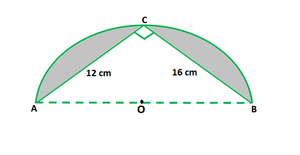Given that,

∆ACB is a right-angled triangle, in which AC = 12 cm, BC = 16 cm, ∠C = 90°

Now In ∆ACB,

By using Pythagoras theorem, we get

AB2 = AC2 + BC2

AB2 = 122 + 162

AB2 = 144 + 256

AB2 = 400

AB = √400

AB = 20 cm

So, the diameter of a semicircle = 20 cm

So, the radius of semicircle, r = 10 cm

Now we find the perimeter of a Shaded region = circumference of semicircle + AC + AB

= πr + 12 + 16

= 3.142 × 10 + 28

= 31.42 + 28

= 59.42 cm

Now we find the area of the shaded region = Area of a semicircle – Area of a right angle triangle

= 1/2  πr2 – 1/2 × base × height

= 1/2 × 3.142 × 102 – 1/2 × AC × BC

= 1/2  × 3.142 × 100 – 1/2 × 12 × 16

= 3.142 × 50 – 6 × 16

= 157.1 – 96

= 61.1 cm2

Hence, the required Perimeter of a Shaded region is 59.4 cm and area of the shaded region is 61.1 cm2.

### (ii) the area of the shaded region.

Solution: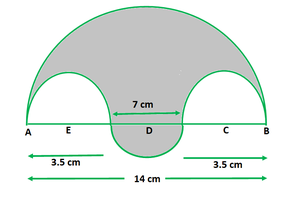Given that,

The shaded region consists of four semi-circular arcs,

the smallest two being equal.

If the diameter of the largest is 14 cm and of the smallest is 3.5 cm.

(i) Length of the boundary = (boundary of bigger semicircle +

boundary of smaller semicircle +

2 × boundary of smallest semicircle)

= π(14/2) + π(7/2) + 2× π(3.5/2)

= 7π + 3.5π + 3.5π

= 7π + 7π

= 14π

= 14 × 22/7

= 2 × 22

= 44 cm

Hence, the length of the boundary = 44 cm

(ii) Now we find the area of the shaded region = Area of semicircle with AB as diameter –

Area of the semicircle with radius AE –

Area of the semicircle with radius BC +

Area of semicircle with diameter 7 cm

= 1/2 × π(14/2)2 – 1/2 × π(3.5/2)2 – 1/2 × π(3.5/2)2 + 1/2 × π(7/2)2

= 1/2π [72 – 1.752 – 1.752 + 3.52]

= 1/2 π[49 – 3.0625 – 3.0625 + 12.25]

= 1/2 π[49 – 6.125 + 12.25]

= 1/2 π [42.875 + 12.25]

= 1/2 π [55.125]

= 1/2 × 22/7 × 55.125

= 11 × 7.875

= 86.625 cm2

Hence, the required area of the shaded region is 86.625 cm2

### Question 43. In the figure, AB = 36 cm and M is mid-point of AB. Semi-circles are drawn on AB, AM, and MB as diameters. A circle with centre C touches all the three circles. Find the area of the shaded region.

Solution: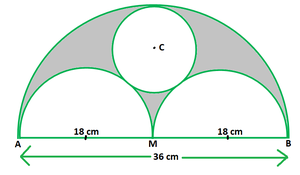Given that,

AB = 36 cm

AM = BM = 1/2 × AB = 1/2 × 36 = 18 cm [M is mid-point of AB]

AM = BM = 18 cm

AP = PM = MQ = QB = 9 cm

Let us considered the radius of circle with centre C be ‘r’ i.e CR = r

Join P to C and M to C, MC ⊥ AB

MR = AM = 18 cm

CM = MR – CR

CM = (18 – r )………(1)

PC = PE + CE

PC = (9 + r)…….(2)

Now In ∆ PCM,

By using Pythagoras theorem, we get

PC2 = PM2 + MC2

(9 + r)2 = 92 + (18 – r)2

81 + r2 + 18r = 81 + 324 + r2 – 36r         [From eq (1) and (2)]

54r = 324

r = 324/54

r = 6

Radius of circle with C as a centre = 6 cm

Now we find the area of shaded region = Area of semicircle with diameter AB –

Area to semicircles with diameter AM and MB –

Area of circle with C as a centre

= 1/2 π(36/2)2 – 2 × 1/2 π(18/2)2 – π(6)2

= 1/2 π(18)2 – π(9)2 – π(6)2

= 1/2 π × 324 – 81π – 36π

= 162π – 81π – 36π

= 162π – 117π

= 45π cm2

Hence, the area of required shaded region is 45π cm2.

### Question 44. In the figure, ABC is a right-angled triangle in which ∠A = 90°, AB = 21 cm and AC = 28 cm. Semi-circles are described on AB, BC, and AC as diameters. Find the area of the shaded region.

Solution: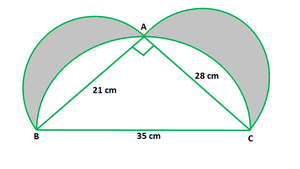Given that,

ABC is a right-angled triangle in which ∠A = 90°, AB = 21 cm and AC = 28 cm.

To find: the area of the shaded region.

Now In right ΔABC,

By using Pythagoras theorem, we get

BC2 = AB2 + AC2

BC2 = 212 + 282

BC2 = 1225

BC = √1225

BC = 35 cm

Diameter BC = 35 cm

Now we find the area of shaded region, A = Area of semicircle with AC as a diameter +

Area of right angle ∆ ABC +

Area of semicircle with AB as a diameter  –

Area of semicircle with BC as diameter

= 1/2 π(21/2)2 + 1/2 π(28/2)2 + 1/2 × 21 × 28 – 1/2 π(35/2)2

= 1/2 π(21/2)2 + 1/2 π(28/2)2 – 1/2 π(35/2)² + 1/2 × 21 × 28

= 1/2 π [10.52 + 142 – 17.52] + 14 × 21

= 1/2 π [110.25 + 196  – 306.25] × 294

= 1/2 π [306.25 – 306.25] + 294

= 1/2 π × 0 + 294

= 0 + 294

= 294 cm2

Hence, the area of required shaded region is 294 cm2

### (iii) the area of the cross-section.

Solution: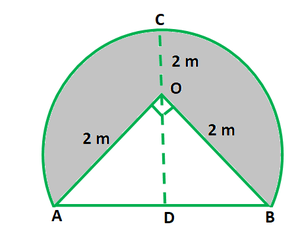Given that,

The radius OA of the circular part = 2 m

∠AOB = 90°

Let OM ⊥ AB.

(i) Now In ∆OAB,

By using Pythagoras Theorem, we get

AB2 = OA2 + OB2

AB2 = 22 + 22

AB2 = 8

AB = √8

AB = √4×2

AB = 2√2 cm

Here, D b e the mid point so, AD = BD = √2

So, OD2 = OA2 – AD2

= 22 – (√22)

= √2

Let the height of the tunnel to be h.

So,

The area of ∆ OAB = 1/2 × Base × height

= 1/2 × OA × OB

1/2 × 2 × 2

= 2

(i) Height of the tunnel (h) = OC + OD

h = √2 + 2

h = (2 + √2)m

(ii) Central angle of major arc, θ = 360° – 90° = 270°

Perimeter of cross-section,

= length of the major Arc AB + AB

= θ/360° × 2πr + 2√2

= 270°/360° × 2π × 2 + 2√2

= 3/4 × 4π + 2√2

= (3π + 2√2) m

(iii) Area of cross-section, A = θ/360° × Area of circle + area of ∆AOB

= θ/360° × πr2 + 1/2 × base × height

= 270°/360° × π× 22 + 1/2 × 2 × 2

= 3/4 × π × 4 + 2

= (3π + 2)m

Hence, the height of the tunnel is  (2 + √2)m,

Perimeter of cross-section is (3π + 2√2) m and

Area of cross section is (3π + 2)m.

### Question 46. In the figure, shows a kite in which BCD is the shape of a quadrant of a circle of radius 42 cm. ABCD is a square and ΔCEF is an isosceles right angled triangle whose equal sides are 6 cm long. Find the area of the shaded region.

Solution: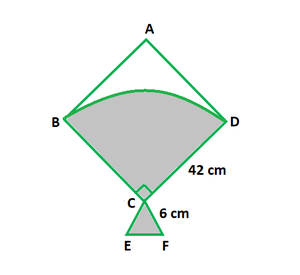Given :

Equal sides of an isosceles right-angled ∆ = 6 cm

To find: the area of the shaded region.

Now we find the area of shaded region(A) = Area of quadrant + Area of isosceles ∆

A = 1/4 πr2 + 1/2 × base × height

A = 1/4 × 22/7 × 422 + 1/2 × 6 × 6

A = 1/2 × 11 × 6 × 42 + 18

A = 11 × 3 × 42 + 18

A = 33 × 42 + 18

A = 1386 + 18

A = 1404 cm2

Hence, the area of shaded region is 1404 cm2

### Question 47. In the figure, ABCD is a trapezium of area 24.5 cm2. In it, AD || BC, ∠DAB = 90°, AD = 10 cm and BC = 4 cm. If ABE is a quadrant of a circle, find the area of the shaded region. (Take π = (22/7).

Solution: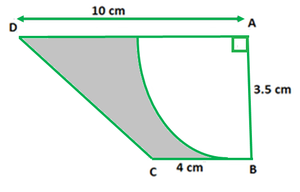Given,

Area of trapezium ABCD, A = 24.5 cm2

AD || BC, ∠DAB = 90°, AD = 10 cm, BC = 4 cm and ABE is quadrant of a circle.

Now in trapezium ABCD,

Area of the trapezium, A = 1/2 (sum of parallel sides) × perpendicular distance between the parallel sides(h)

A = 1/2 (AD + BC) × AB

24.5 = 1/2 (10 + 4) × AB

24.5 × 2 = 14 AB

AB = 49/14

AB = 7/2

AB = 3.5 cm

So, the radius of the quadrant of the circle, r = AB = 3.5 cm

Area of the quadrant of the circle = 1/4 ×πr2

= (1/4) (22/7 x 3.5 x 3.5)

= 9.625 cm2

Now we find the area of the shaded region = Area of the trapezium – Area of the quadrant of the circle

= 24.5 – 9.625

= 14.875 cm2

Hence, the area of the shaded region is 14.875 cm2

### Question 48. In the figure, ABCD is a trapezium with AB || DC, AB = 18 cm, DC = 32 cm and the distance between AB and DC is 14 cm. Circles of equal radii 7 cm with centres A, B, C, and D have been drawn. Then, find the area of the shaded region of the figure. (Use π = 22/7).

Solution: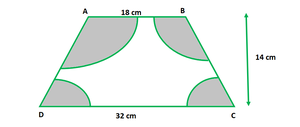Given,

AB = 18 cm, DC = 32 cm,

Distance between AB and DC(h)= 14 cm and radius of each circle(r) = 7cm

Since, AB ||DC

So, ∠A + ∠D = 180° & ∠B + ∠C = 180°

Area of sector = (θ /360) × πr2

Area of sector with ∠A and ∠D = (180 /360) × 22/7 × 72

= 1/2 × 22 × 7 = 11 × 7 = 77 cm2

Similarly,  Area of sector with ∠B & ∠C = (180 /360) × 22/7 × 72

= 1/2 × 22 × 7 = 11 × 7 = 77 cm2

Now in trapezium ABCD,

Area of trapezium = 1/2 (sum of parallel sides) × perpendicular distance between Parallel sides(h)

= 1/2 (AB + DC) × (h)

= 1/2(18 + 32) × 14

= 1/2(50)× 14

= 25 × 14 = 350 cm2

Now we find the area of shaded region = Area of trapezium –

(Area of sector with ∠B and ∠C +

Area of sector with ∠A and ∠D )

= 350 -(77+77) = 350 – 154 = 196 cm2

Hence, the Area of shaded region is 196 cm2

### Question 49. From a thin metallic piece, in the shape of a trapezium ABCD, in which AB || CD and ∠BCD = 90°, a quarter circle BEFC is removed (see figure). Given AB = BC = 3.5 cm and DE = 2 cm, calculate the area of the remaining piece of the metal sheet.

Solution: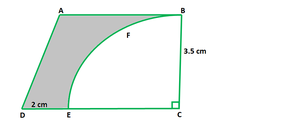Given,

In trapezium ABCD

AB || CD and ∠BCD = 90°

AB = BC =3.5 cm & DE = 2 cm

CE = CB = 3.5 cm [CE and BC are the radii of quarter circle BFEC]

So, DC = DE + EC

DC = 2 cm + 3.5 cm

DC = 5.5 cm

Area of remaining piece of the metal sheet (A) = Area of trapezium ABCD – Area of quarter circle BFEC

A = 1/2(AB + DC) × BC – 1/4 x π x (BC)2

A = 1/2 (3.5 + 5.5) × 3.5  – 1/4 x π(3.5)2

A = 1/2 × 9  × 3.5 – 1/4 x π(3.5)2

A = 4.5 × 3.5  – 22/7 × 3.5 × 3.5/4

A = 15.75 –   11 × 3.5/4

A = 15.75 – 9.625

A = 6.125 cm2

Hence, the area of remaining piece of the metal sheet (Shaded region) is 6.125 cm2

### Question 50. In the figure, ABC is an equilateral triangle of side 8 cm. A, B and C are the centres of circular arcs of radius 4 cm. Find the area of the shaded region correct upto 2 decimal places. (Take π = 3.142 and √3 = 1.732).

Solution: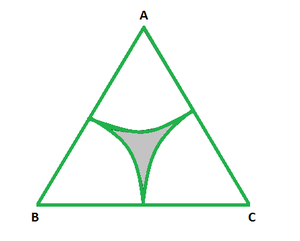In Equilateral triangle all the angles are each 60°.

The corners form sectors of a circle.

When we join the sectors we form a major sector with the middle angle as (60 × 3) = 180°

Area of the shaded region = Area of the triangle – area of the sector.

Area of the triangle = 1/2 × base × height

As we know that,

Base = 8/2 = 4 cm

Hypotenuse = 8 cm

Height = √82 – 42

= √48

= 4√3

= 4 × 1.732

= 6.928 cm

Also, area of the triangle = 1/2 × 6.928 × 8 = 27.712 cm2

Now area of the Sector,

Radius of the sector = 8/2 = 4 cm

= 180/360 × 3.142 × 42 = 25.136 cm2

Now we find the area of the shaded region = 27.712 – 25.136 = 2.576 cm2

Hence, the area of the shaded region is 2.576 cm2

### Question 51. Sides of a triangular field are 15 m, 16 m, and 17 m. With the three corners of the field a cow, a buffalo and a horse are tied separately with ropes of length 7 m each to graze in the field. Find the area of the field which cannot be grazed by three animals.

Solution: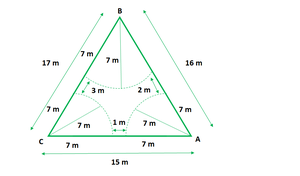Let ABC be the triangular field with sides AC = 15 m, AB = 16 m and BC = 17 m

And,

Let the place where the buffalo, the horse and the cow are tied,

are three sectors i.e. sector BFG, sector CHI and sector ADE

Area of triangular field = √s(s – a)(s – b)(s – c)   [by using Heron’s formula]

s = (a + b + c)/2

s = (15 + 16 + 17)/2

s = 48/2

s = 24 m

=√24(24 – 15)(24 – 16)(24 – 17)

=√24 x 9 x 8 x 7

=√12096

=109.98 m2

Area of triangular field = 109.98 m2

Area of the grazed part = Area of the sector ADE + Area of sector BFG + Area of sector CHI

= π x 72 x ∠A/360 + π x 72 x ∠B/360 + π x 72 x ∠C/360

= π x 72(∠ A + ∠ B + ∠ C)/360

= 22/7 x (7)2 x 180/360

= 154/2

= 77 m2

So, the area of the field which cannot be grazed by these animals

= 109.98 m2 – 77 m2

= 32.98 m2

Hence, the area of the field which cannot be grazed by these animals is 32.98 m2

### Question 52. In the given figure, the side of a square is 28 cm, and radius of each circle is half of the length of the side of the square where O and O’ are centres of the circles. Find the area of shaded region.

Solution: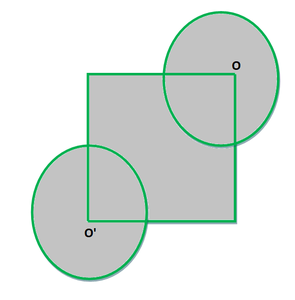Given that,

Side of square = 28 cm

Radius of each circle is half of the length of the side of the square

So, radius of each circle = 28/2 cm =14 cm

As we know that

Area of Square = (Side)2

Area of Circle = πr2

Now we find the area of Shaded region = Area of Square +3/4 (Area of Circle) + 3/4(Area of Circle)

= (28)2 + 3/2 x 22/7 × 14 × 14

= 784 cm2 + 924 cm2

= 1708 cm2

Hence, the area of shaded region is 1708 cm2

### Question 53. In a hospital used water is collected in a cylindrical tank of diameter 2 m and height 5 m. After recycling, this water is used to irrigate a park to hospital whose length is 25 m and breadth is 20 m. If tank is filled completely then what will be the height of standing water used for irrigating the park?

Solution:

Given that

Diameter of cylinder (d) = 2 m

Radius of cylinder (r) = 1 m

Height of cylinder (H) = 5 m

Now we know that volume of cylindrical tank is,

V = πr2H = π × (1)2 × 5 = 5π m

Length of the park (l) = 25 m

Breadth of park (b) = 20 m

Let us considered the height of standing water in the park = h

Volume of water in the park = l x b x h = 25 × 20 × h

Now for irrigation in the park water is used from the tank. So,

Volume of cylindrical tank = Volume of water in the park

⇒ 5π = 25 × 20 × h

⇒ 5π/25 × 20 = h

⇒ h = π/100 m

⇒ h = 0.0314 m

Hence, the height of standing water used for irrigating the park is 0.0314 m

My Personal Notes arrow_drop_up